Effect of Ten CFD Numerical Schemes on Repeated Richardson Extrapolation (RRE) | OMICS International
Journal of Applied & Computational Mathematics

# Effect of Ten CFD Numerical Schemes on Repeated Richardson Extrapolation (RRE)

Carlos Henrique Marchi* and Eduardo Matos Germer

Federal University of Paraná (UFPR) Department of Mechanical Engineering Curitiba, PR, Brazil

*Corresponding Author:
Carlos Henrique Marchi
Federal University of Paraná (UFPR)
Department of Mechanical Engineering Curitiba, PR, Brazil
Tel: 554133613126
Fax: 554133613701
E-mail: [email protected]

Received March 20, 2013; Accepted May 22, 2013; Published May 30, 2013

Citation: Marchi CH, Germer EM (2013) Effect of Ten CFD Numerical Schemes on Repeated Richardson Extrapolation (RRE). J Appl Computat Math 2:128. doi: 10.4172/2168-9679.1000128

Copyright: © 2013 Marchi CH, et al. This is an open-access article distributed under the terms of the Creative Commons Attribution License, which permits unrestricted use, distribution, and reproduction in any medium, provided the original author and source are credited.

Visit for more related articles at Journal of Applied & Computational Mathematics

#### Abstract

The main objective of this work is to evaluate the performance of RRE in reducing the discretization error when associated with ten types of CFD numerical schemes of first, second and third orders of accuracy. The onedimensional advection-diffusion equation is solved with the finite volume method, for five values of the Peclet number (Pe), with uniform grids of 5 to 23,914,845 volumes, allowing for up to 14 RRE. Results are obtained for temperature at the center of the domain, average of the temperature field, and heat transfer rate. It was found that: (1) RRE is extremely effective in reducing the discretization error for all the variables, numerical schemes and Pe, reaching an order of accuracy of up to 18.9; and (2) The second-order central difference scheme together with RRE is the one that presents the smallest error for the dependent variable.

#### Keywords

Richardson extrapolation; Advection-diffusion equation; High-order scheme; Discretization error; Order of accuracy; Verification

#### Introduction

The Repeated Richardson Extrapolation (RRE) was created [1,2] for the purpose of reducing and estimating the discretization error  of numerical solutions. To use RRE requires having the numerical solution of the variable of interest in three or more grids with different numbers of nodes, obtained by the finite volume or finite difference methods, for example. The importance of RRE in the area of CFD (Computational Fluid Dynamics) can be understood upon analyzing the optimal results of works [4-7]. For example, the minimum value of the stream function obtained in  was -0.11894 with two Richardson extrapolations based on 100x100, 120x120 and 140x140 grids. This result refers to the solution of the 2D Navier-Stokes equations of the lid-driven square cavity problem for the Reynolds number 1,000. This result has a smaller error than that obtained on a 1024x1024 grid without extrapolation. The above cited works solved two-dimensional problems using only the central difference scheme of second-order accuracy with up to 9 RRE. The following questions remain unanswered in the current literature:

a) It is known that without RRE, the higher the order of accuracy of the scheme the smaller the discretization error of the dependent variable for the same sufficiently fine grid. Could this also be the case when the schemes are associated with RRE?

b) Does any scheme, even of first-order accuracy, significantly reduce the discretization error when associated with RRE?

c) Can two schemes with the same order of accuracy result in very different discretization errors when associated with RRE?

d) Does the solution of advection-diffusion problems together with RRE result in reductions of the discretization error as large as in purely diffusive problems such as those obtained in [8,9]?

e) What is the effect of the various schemes together with RRE on the reduction of the discretization error of secondary variables, which are obtained by additional numerical approximations on the solution of the dependent (primary) variable?

f) What is the Peclet number effect on the reduction of the error of a scheme together with RRE?

To answer the above questions this study tests, along with RRE, ten types of schemes of first, second and third orders of accuracy , almost all of which are widely used in CFD. Grids with up to millions of nodes are used, resulting in up to fourteen RRE. For five values of the Peclet number, results are obtained for the temperature at the center of the calculation domain (dependent variable) and two secondary variables: average of the temperature field and the heat transfer rate. Finding the answers to these questions is irrefutable evidence of the importance of this work, because knowing what the best combination of a numerical scheme with RRE is enables one to obtain numerical solutions to practical problems of CFD with smaller error and at lower computational cost (RAM and CPU time).

Obviously, to perform this work, the ideal situation would be to solve three-dimensional or at least two-dimensional problems. However, as we will see in the results section, this could not be done with the computers available today, i.e., use millions of nodes in each direction; such a grid size would be required to adequately characterize the error and its orders of variables with the various schemes, associated or not with the RRE. Therefore, in this work, a one-dimensional advectiondiffusion problem is solved using the finite volume method on uniform grids. Nevertheless, RRE can be used in two-dimensional problems when using coarser grids and fewer REs than in the present work, as was done in [4-9]. Although RRE can also be used in three-dimensional problems, we are unaware of studies that have done this. Preliminary results of this work have been published in . A complete and detailed description of this work can be found in .

#### Mathematical Model

The mathematical model considered in this work consists of the one-dimensional steady state advection-diffusion equation with Dirichlet boundary conditions. This equation is widely used in testing new numerical models  and is defined by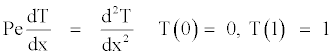(1)

where Pe is the Peclet number, x is the coordinate direction, and T is the temperature. The analytical solution of Eq. (1) is T(x)=(exp(xPe)-1)/ (exp(Pe)-1). The variables of interest are: (1) the temperature at the center of the domain, i.e., at x=½, represented by Tc; (2) the average temperature field, represented by Tm; (3) the heat transfer rate at x=1, represented by q; and (4) the average of the l1 norm of the discretization error of T, represented by L. The variable Tc was chosen because it is the dependent variable in the differential equation of the problem, i.e., the primary variable of the problem. The variables Tm and q were chosen because they represent secondary variables, i.e., calculated with numerical approximations in addition to those used to obtain T. The variable L was chosen because it allows the discretization error of the entire field of T to be represented with a single value; its definition is .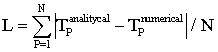The variables Tm and q are defined mathematically by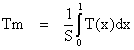,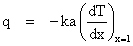(2)

where S=1 m, k=1 W/m.K and a=1 m2 represent, respectively, the length of the calculation domain, the thermal conductivity, and the area of heat exchange.

#### Numerical Models

Numerical solutions without extrapolation

With the finite volume method , integrating Eq. (1) on the generic control volume P of Figure 1, one obtains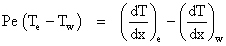(3)

Figure 1: Uniform 1D grid of the center node type with the generic P volume and its W and E neighbors.

To approximate T on the west (w) and east (e) faces of each P volume, which is required in Eq. (3), the following advection schemes were used, indicated together with their orders of accuracy:

• UDS-1: Upwind Differencing Scheme (first order) 

• Alpha (first order) 

• CDS-2: Central Differencing Scheme (second order) 

• UDS-2: Upwind Differencing Scheme (second order) 

• QUICK: Quadratic Upstream Interpolation for Convective Kinematics (third order) [10,12]

• TVD: Total Variation Diminishing (Superbee type, second order) .

To approximate the first-order derivative of T on the west (w) and east (e) faces of each P volume, which is required in Eq. (3), the following diffusion schemes were used, indicated together with their orders of accuracy:

• CDS-2: Central Differencing Scheme (second order) 

• CDS-4: Central Differencing Scheme (fourth order). In this case, four types of third-order schemes were also used to evaluate the firstorder derivatives of Eq. (3) on the faces of the two boundaries and on the face closest to each boundary, to which the CDS-4 scheme cannot be applied . To approximate T and its first-order derivative on the west (w) and east (e) faces of each P volume, which is required in Eq. (3), the following advection-diffusion schemes were used, indicated together with their orders of accuracy:

• WUDS: Upstream-Weighted Differencing Scheme (second order) 

• PLDS: Power-Law Differencing Scheme (second order) .

Using the seven advection schemes and two diffusion schemes mentioned above, eight advection-diffusion schemes were created. These, together with the WUDS and PLDS, resulted in the ten advection-diffusion schemes tested in this work, which are listed in Table 1. This table also presents the value expected for the asymptotic order or order of accuracy (p0) of the discretization error of the solution of T. These orders were obtained through a priori analyses according to the procedure described in  for the finite volume method and the type of grid shown in Figure 1. Hereafter, each advection-diffusion scheme will be referred to by its acronym listed in Table 1. The way in which the UDS1, UDS2, CDS, PLDS and QC2 schemes were used in the present work can be seen in ; the WUDS, TVD and ADS schemes in ; the Alpha scheme in ; and the QC3 scheme in . The latter reference also gives a complete and detailed description of the application of the ten schemes, including the coefficients and independent terms of the systems of equations, for both the real and ghost control volumes that were used to apply the boundary conditions; and the a priori analyses performed to obtain p0. After applying each of the ten advection-diffusion schemes on Eq. (3), one obtains a system of algebraic equations of the [A][T]=[B] type, where [A] is the matrix of coefficients, [T] is the unknown vector, and [B] is the vector of the independent terms. The TDMA (TriDiagonal Matrix Algorithm) method  was used to solve the systems of algebraic equations. For the UDS1, Alpha, CDS, WUDS and PLDS schemes, the TDMA solves the systems directly, without iterations. In the case of the other five schemes (UDS2, ADS, TVD, QC2 and QC3), the solution is iterative. In this work, the numerical solution in each grid, obtained by the TDMA method as described above, is called a solution without extrapolation or without RRE. The numerical solution of the variable Tc is obtained directly from the central node of each grid after the numerical solution of Eq. (1); this is possible because grids with an odd number of nodes were used. The numerical solution of Eq. (2) for Tm was obtained by numerical integration by the rectangle rule  (second order of accuracy). The numerical solution of q was obtained by means of the UDS-2 scheme (second-order Upwind Differencing Scheme) to evaluate the derivative of Eq. (2). Details of the calculation of Tm, q and L are given in .

Acronym Advection Diffusion Asymptotic order p0
UDS1 UDS-1 CDS-2 1
Alpha Alpha CDS-2 1
CDS CDS-2 CDS-2 2
UDS2 UDS-2 CDS-2 2
WUDS WUDS WUDS 2
PLDS PLDS PLDS 2
TVD TVD CDS-2 2
QC2 QUICK CDS-2 2
QC3 QUICK CDS-4 and others-3 3

Table 1: The 10 advection-diffusion schemes tested in Eq. (1).

Numerical solutions with repeated Richardson extrapolation (RRE)

The numerical solution without extrapolation is obtained as described above for a set of grids g=[1,G], where g=1 is the coarsest grid, i.e., the one with the largest h size of the control volumes in Figure 1, and g=G is the finest grid, i.e., the one with the smallest h size of the control volumes in Figure 1. For each variable of interest (Ø), its numerical solution in grid g with m Richardson extrapolations is given by [8,9]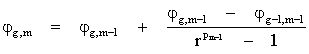(4)

Where r=hg-1/hg is the grid refinement ratio, and the variable pm represents the true orders  of the discretization error. Equation (4) is valid for g=[2,G] and m=[1,g-1]. In the present work, the variable Ø represents each of the variables of interest already defined in section 2, i.e., Tc, Tm and q. It should be noted that to obtain each value of Øg,m requires having two numerical solutions Ø on two grids (g and g-1) at m-1. For any g, m=0 represents the numerical solution of Ø without any extrapolation, obtained as described above. For m=1, one has the standard or simple Richardson extrapolation. For a given value of g, Eq. (4) can be applied up to g-1 times, performing a number of Richardson extrapolations equal to m=g-1.

#### Results

The numerical solutions without and with extrapolation were obtained, respectively, using the computational programs Peclet 1Dp 2.2 and Richardson 3.1. Both these programs were implemented in Fortran 95 (Intel 9.1) language and quadruple precision (Real*16); they are available at the web site ftp://ftp.demec.ufpr.br/CFD/projetos/ cfd4/. The simulations were performed in a core of an Intel Core 2 Quad processor inserted in a computer with a clock speed of 2.4 GHz, 8 GB of RAM and Windows XP 64 bit operating system. For each of the ten schemes, solutions were obtained in G=15 grids with N=5, 15, 45, 135, 405, 1,215, 3,645, 10,935, 32,805, 98,415, 295,245, 885,735, 2,657,205,7,971,615 and 23,914,845 real volumes; hence, the grid refinement ratio is r=3. This ratio was used to enable Tc to be obtained in the various grids, without any numerical approximation besides those employed to solve Eq. (1). The Alpha coefficient=0.05 was used for the Alpha scheme. In the case of the UDS2, ADS, TVD, QC2 and QC3 schemes, the solution is iterative. For these schemes, the iterative process was performed until the machine round-off error was reached, aiming to reduce the impact of the iteration error on the numerical error as much as possible. In all the calculations, for the 10 schemes and all the variables of interest, quadruple precision (Real*16) was used, aiming to reduce to a minimum the impact of the round-off error on the numerical error. As shown in [8,9], with double precision (Real*8) and RRE, the numerical error is already affected by the round-off error on grids with more than 100 nodes in each spatial direction. In addition, several tests were performed to reduce to a minimum the possible occurrence of a programming error. With these precautions, the main source of numerical error  is the discretization error, which can then by measured by means of 

E(Ø)=Ø -Φ (5)

where Ø and Φ represent, respectively, the numerical and exact analytical solutions of each variable of interest. To measure the numerical error with Eq. (5), the analytical solution (Φ) of each variable of interest was obtained using Maple software with 64 digits. For the ten schemes on the same grid (N), the ratio between the largest and the smallest computational memory needed to solve the problem varied from 1.28 to 1.12, respectively, for the coarsest and the finest grid. Therefore, the effect of the schemes on the memory is not very relevant and decreases as the grid becomes more refined. As for the CPU time required to solve the problem, the effect can be significant. Among the five non-iterative schemes (UDS1, Alpha, CDS, WUDS and PLDS), the ratio between the longest and the shortest CPU time needed to solve the problem is on average 1.21, considering the fifteen grids used. However, among the five iterative schemes (UDS2, ADS, TVD, QC2 and QC3), on the same grid (N) and for the same convergence criterion, the ratio between the shortest CPU time of the iterative schemes and the shortest CPU time of the non-iterative schemes is equal to at least 1.87 and at most 4.35, depending on N. And the ratio between the longest CPU time of the iterative schemes and the shortest CPU time of the non-iterative ones is equal to at least 6.90 and at most 19.7, depending on N. Due to space restrictions, the sections below present only a summary of the results. However, additional results can be seen in , and all the results of this work are described in . This reference contains results of orders of accuracy and errors with and without RRE for all the variables, schemes and Pe, as well as for Pe=100.

True orders of the discretization error

Table 2 presents the true orders (pT)  of the discretization error of the four variables of interest and ten schemes in Table 1, obtained for Pe=5. In each column, for each variable of interest, the ten schemes are distributed according to the values obtained for pT. The values of pT. are the exponents of equation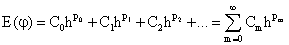, where Cm are coefficients that depend on Φ and its derivatives. These orders were obtained by a posteriori analyses according to the procedure described in [8,9], which is based only on the numerical solution of each variable of interest on several grids; the analytical solution does not need to be known. The correct determination of pT is essential to obtain the best performance of RRE. The asymptotic order or order of accuracy (p0) is the lowest of the pT values of each sequence shown in Table 2 for each scheme and variable. For the variable Tc, note that all the a posteriori results of p0 (Table 2) coincide with the a priori results (Table 1). Additional comments about the results in Table 2 are given in sections 4.2 and 4.3. Figure 2 shows the results of the apparent orders (pU) [8,9] of the estimated discretization error of the variable Tc, obtained with the QC3 scheme and Pe=5, as a function of the size (h) of the control volumes (Figure 1), where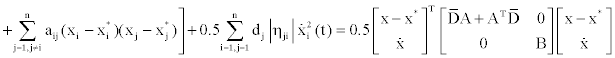The pT value of each level of extrapolation m is characterized when one verifies the tendency of pU to h→0. It is clear, in Figure 2, that p0=pT(m=0) = 3; and that p1=pT(m=1)=4. Furthermore, p2=pT(m=2) seems to tend toward 5. In general, the proper use of RRE requires simply determining pT of m=0 and 1. At the lowest value of h on the m=0 curve, the distance between the value 3 and pU increases due to round-off errors; this also affects the two lower values of h on the m=1 curve. Figure 2 also lacks some values of pU due to the calculation process and larger round-off errors.

Tc e L Tm q pT
UDS1, Alpha UDS1, Alpha UDS1, CDS, UDS2, WUDS, PLDS, ADS, TVD 1, 2, 3, ...
UDS2, WUDS, PLDS, ADS, QC2 UDS2, WUDS, PLDS, ADS, QC2, QC3 QC2, QC3 2, 3, 4, ...
CDS, TVD CDS, TVD --- 2, 4, 6, ...
QC3 --- --- 3, 4, 5, ...

Table 2: True orders (pT) of the discretization error of the variables of interest.

Errors and their primary variable orders

Figure 3 shows the error modulus (E) of the numerical solution of the variable Tc vs. h for Pe=5. Eh and Em represent, respectively, results without and with RRE; in other words, Eh refers to the error of numerical solutions without extrapolation, obtained as described previously; and Em refers to the error of numerical solutions with repeated Richardson extrapolation (RRE), obtained as described previously. Figure 3 and Figures 4-6 show only four schemes that represent the main results to be highlighted. With regard to the results of the ten schemes without extrapolation (Eh) of Tc, it was found that:

• The UDS1 and Alpha schemes are first-order accurate.

• The UDS2, CDS, WUDS, PLDS, ADS, TVD and QC2 schemes are second-order accurate.

• The QC3 scheme is third-order accurate.

• The three results above were expected according to their values of p0 in Table 1.

• For the same h and h ≤2.2x10-2, i.e., with N≥45 nodes, the UDS1 and QC3 schemes have, respectively, the largest and the smallest error among the ten schemes.

• None of the schemes reach the minimum error of quadruple precision, even at the lowest h=4.2x10-8 or N=23,914,845 nodes. Therefore, the results of Eh of the ten schemes for Tc are in accordance with the known literature. This increases the reliability of the results obtained with RRE, according to the above results. As for the results of the ten schemes with extrapolation (Em) of Tc, it was found that:

• For 3.4x10-6 ≤ h ≤ 3.0x10-5, i.e., with N between 32,805 and 295,245 nodes, all the ten schemes reach the minimum level of error of quadruple precision. For lower values of h, the round-off error becomes larger than the discretization error, which results in an increase in the numerical error with the reduction of h.

• For the same h and h ≤ 7.4x10-3, i.e., with N ≥ 135 nodes, the UDS1 and CDS schemes have, respectively, the largest and the smallest error among the ten schemes, while the discretization error prevails over the numerical error.

• For the same h and h ≤ 8.2x10-4, i.e., with N ≥ 1,215 nodes, the TVD scheme has the second smallest error among the ten schemes, while the discretization error prevails over the numerical error.

• Except for the UDS1 and CDS schemes, the other eight schemes generally present crossovers among the various Em vs. h curves.

Here we have an unexpected result that is unknown in the literature: except in the first extrapolation (whose h ≈ 6.7x10-2 and N=15 nodes in Figure 3), the second-order CDS scheme presents a smaller error than the third-order QC3 scheme, when both use RRE type extrapolation. The probable explanation for this is that, already in the second extrapolation (whose h ≈ 2.2x10-2 and N=45 nodes in Figure 3), the theoretical order of the extrapolation of the CDS scheme is already six, while that of the QC3 scheme is five. As can be seen in Table 2, at each new extrapolation, the order of the CDS scheme is augmented by two units while that of the QC3 scheme increases by only one unit, increasing the difference between the error curves at each additional extrapolation. Similarly, for h ≤ 8.2x10-4, the second-order TVD scheme presents a smaller error than the third-order QC3 scheme, when both use RRE. A comparison of the Eh and Em curves for Tc in Figure 3 clearly indicates that the use of RRE is extremely efficient in reducing the discretization error of the primary variable. This error reduction is greater the lower h is. Even the UDS1 scheme with RRE (which has the largest error among the schemes with RRE) assumed a smaller error than that of the most elaborate scheme without RRE, QC3 (whose error is the smallest among the schemes without RRE), on grids with h ≤8.2x10-4 or N ≥ 1,215 nodes. The results of Eh for variable L are qualitatively equal to those of variable Tc. Therefore, the Eh curves in Figure 3 also qualitatively represent the results of variable L. The results of L are important in order to have an evaluation of all the nodes on each grid with a single parameter. Table 2 shows the value of p0 of all the ten schemes applied to the variable L. Figure 4 shows the effective orders (pE)  of the error curves in Figure 3, i.e., of variable Tc. Figure 4 does not show the results of the curves of Figure 3 whose numerical error is dominated by the round-off error, because the concept of pE is valid for the discretization error. The maximum value of pE obtained by each scheme with RRE is: UDS1=11.0, QC2=12.8, CDS=13.7 and QC3=11.0. It should be kept in mind that the pE of these schemes without RRE is: UDS1=1; QC2=CDS=2; and QC3=3. Therefore, it is clear that there is a significant increase in the order of the discretization error in all the schemes with RRE. As can be seen in Figure 4, on average, the slopes of the curves of the UDS1, QC2 and QC3 schemes are smaller than that of the curve of the CDS scheme. This is because the first three schemes present a variation equal to unity in their true orders, while this variation in the CDS scheme is equal to two. Tables 3 and 4 help illustrate the effect of the use of RRE in reducing the error for the variable Tc. For three specific error levels, Table 3 shows the grid required to obtain the same error without and with RRE. For example, for the error level of 1E-10, without RRE, a grid with 98,415 nodes must be used to reach approximately this error level, and a grid with 135 nodes but with three extrapolations; hence, with RRE one requires a grid with 729 times fewer nodes. This ratio between the number of nodes in the Eh and Em grids indicates the level of reduction of the computational cost (memory and CPU time) afforded by the use of RRE when compared to not using it. Table 3 shows that the smaller the error level the better the performance of RRE. For three specific grids, Table 4 shows the effect of the reduction of the discretization error when using RRE, measured by the |Eh|/|Em| ratio. For example, for a grid with 45 nodes, even with only two extrapolations, the error with RRE is already reduced by about 2,400 times in relation to the solution without RRE. Table 4 shows that the higher the number of nodes in the grid the better the performance of RRE.

Error level for Eh and Em 1E-6 1E-10 1E-14
N of grid for the error level without RRE (Eh) 1,215 98,415 7,971,615
N of grid for the error level with RRE (Em) 45 135 405
Number of extrapolations (m) for Em 2 3 4
N of Eh / N of Em 27 729 19,683

Table 3: Grids required for three specific Tc errors with CDS and Pe=5.

N of grid 45 1,215 32,805
Error without RRE (|Eh|) for N 3.1E-4 4.2E-7 5.8E-10
Error with RRE (|Em|) for N 1.3E-7 1.6E-21 9.3E-30
Number of extrapolations (m) for Em 2 5 8
|Eh| / |Em| 2.4E3 2.6E14 6.2E19

Table 4: Tc errors with CDS and Pe=5 on three specific grids.

Errors of the secondary variables

Figure 5 shows the error modulus (E) of the numerical solution of variable Tm vs. h for Pe=5, without (Eh) and with (Em) RRE. With regard to the results of the ten schemes without extrapolation (Eh) of Tm, it was found that:

• The UDS1 and Alpha schemes are first-order accurate.

• The UDS2, CDS, WUDS, PLDS, ADS, TVD and QC2 schemes are second-order accurate.

• The order of accuracy of the QC3 scheme degenerated from third to second-order because the error of the rectangle type numerical integration is second-order accurate.

• For the same h and h ≤2.2x10-2, i.e., with N ≥45 nodes, the UDS1 and QC2 schemes have, respectively, the largest and the smallest error among the ten schemes.

• No scheme reached the minimum error level of quadruple precision, even at the lowest h=4.2x10-8 or N=23,914,845 nodes.

Therefore, the results of Eh of the ten schemes for Tm are in accordance with the known literature, considering the p0 (Table 1) of the ten schemes applied to Eq. (1) plus the p0 of the rectangle type numerical integral, which is 2. This increases the reliability of the results obtained with RRE, as indicated in the results above. Table 2 lists the values of p0 and pT of the ten schemes applied to the variable Tm. With regard to the results of the ten schemes with extrapolation (Em) of Tm, we found that:

• For 3.4x10-6h ≤3.0x10-5, i.e., with N between 32,805 and 295,245 nodes, all the ten schemes reach the minimum error level of quadruple precision. For lower values of h, the round-off error becomes larger than the discretization error, which results in an increase of the numerical error with the reduction of h.

• For the same h and h ≤ 2.2x10-2, i.e., with N ≥45 nodes, the UDS1 scheme has the largest error among the ten schemes, while the discretization error prevails over the numerical error.

• For the same h and h ≤ 8.2x10-4, i.e., with N ≥ 1,215 nodes, the TVD scheme has the second smallest error among the ten schemes, while the discretization error prevails over the numerical error.

• For the same h and h ≤ 6.7x10-2, i.e., with N ≥ 15 nodes, the CDS scheme has the smallest error among the ten schemes, while the discretization error prevails over the numerical error.

• Except for the UDS1 and CDS schemes, the other eight schemes generally present crossovers between the various Em vs. h curves.

The probable explanation for the fact that the smallest errors of Tm are those of the TVD and CDS schemes is that these are the only ones that have values of pT=2, 4, 6, ... while the other six second-order schemes present pT=2, 3, 4, ..., as indicated in Table 2. This explanation is also in agreement with that presented for the variable Tc, whose smallest errors occur with the CDS and TVD schemes. Comparing the Eh and Em curves for Tm in Figure 5, it is clear that the use of RRE is extemely efficient in also reducing the discretization error of a secondary variable. This error reduction is greater the lower h is. Even the UDS1 scheme with RRE (whose error is the largest among the schemes with RRE) shifts to a smaller error than that of the QC2 scheme (which has the smallest error among the schemes without RRE), on grids with h ≤ 2.5x10-3 or N ≥ 405 nodes. Figure 6 shows the error modulus (E) of the numerical solution of variable q vs. h for Pe=5, without (Eh) and with (Em) RRE for nine schemes; the Alpha scheme did not work for this variable. With regard to the results of nine schemes without extrapolation (Eh) of q, it was found that:

• The UDS1 scheme is first-order accurate.

• The order of accuracy of the UDS2, CDS, WUDS, PLDS, ADS and TVD schemes degenerated from second to first-order due to the UDS-2 approximation error used in the calculation of the first-order derivative of Eq. (2). For the same reason, the order of accuracy of the QC3 scheme degenerated from third to second-order. These degenerations in order are shown in  for this and other approximations, as well as for the Poisson, advection-diffusion and Burgers equations.

• The QC2 scheme maintained its second-order accuracy.

• For the same h and h ≤ 6.7x10-2, i.e., with N ≥ 15 nodes, the errors of the UDS2, CDS, WUDS, PLDS, ADS and TVD schemes are practically the same; the UDS1 scheme has a slightly smaller error than they do; the error of the QC3 scheme is smaller than that of UDS1; and lastly, the QC2 scheme has the smallest error among the nine schemes.

• None of the schemes reached the minimum error level of quadruple precision, even at the lowest h=4.2x10-8 or N=23,914,845 nodes.

Table 2 lists the values of p0 and pT of nine schemes applied to the variable q. Note that, except for the UDS1 and QC2 schemes, the T nodal orders of all the other schemes degenerated due to an additional numerical approximation used to obtain the secondary variable q. With regard to the results of nine schemes with extrapolation (Em) of q, it was found that:

• For 3.4x10-6 ≤ h ≤ 1.0x10-5, i.e., with N between 98,415 and 295,245 nodes, all the schemes reach the minimum error level of quadruple precision. For lower values of h, the round-off error becomes larger than the discretization error, resulting in an increase of the numerical error with the reduction of h.

• For the same h and h ≤ 2.2x10-2, i.e., with N ≥ 45 nodes, the UDS1 scheme has the largest error among the nine schemes, while the discretization error prevails over the numerical error.

• For the same h and h ≤ 2.2x10-2, i.e., with N ≥ 45 nodes, the QC3 scheme has the second smallest error among the nine schemes, while the discretization error prevails over the numerical error.

• For the same h and h ≤ 6.7x10-2, i.e., with N ≥ 15 nodes, the QC2 scheme has the smallest error among the nine schemes, while the discretization error prevails over the numerical error.

• For the other six schemes, there is no standard or pattern, since crossovers occur among the various Em vs. h curves. The probable explanation for the fact that the smallest errors of q are those of the QC3 and QC2 schemes is that these two are the only ones that have values of pT=2,3,4, ... while the other seven schemes present pT=1, 2, 3, ..., as indicated in Table 2. This explanation also agrees with those presented earlier for variables Tc and Tm, whose smallest errors occur with the CDS and TVD schemes. Comparing the Eh and Em curves for q in Figure 6, it is clear that the use of RRE is extemely efficient in reducing the discretization error also of this other secondary variable. This reduction in the error is greater the lower h is. Even the UDS1 scheme with RRE (whose error is the largest one among the schemes with RRE) assumes a smaller error than that of the QC2 scheme (which has the smallest error among the schemes without RRE), on grids with h ≤ 8.2x10-4, i.e., with N ≥ 1,215 nodes.

Peclet number effect

Figure 7 shows the error modulus (E) vs. h of the numerical solution of variable Tc obtained with CDS for Pe=0.01, 0.1, 1 and 10, without (Eh) and with (Em) RRE. The CDS scheme was chosen for this analysis of the Peclet number effect on RRE because it is the scheme that presented the smallest error for Tc with RRE among the ten schemes tested with Pe=5. With regard to the results without extrapolation (Eh) of Tc shown in Figure 7, it was found that:

• For the same h and h ≤ 2.0x10-1, i.e., with N≥ 5 nodes, the higher the Pe the higher the Eh.

• The minimum error level of quadruple precision is reached for no Pe, even at the lowest h=4.2x10-8 or N=23,914,845 nodes. Therefore, these results of Eh are in line with the known literature. This increases the reliability of the results obtained with RRE, based on the above results. With regard to the results with extrapolation (Em) of Tc shown in Figure 7, it was found that:

• For the same h and h ≤ 6.7x10-2, i.e., with N ≥ 15 nodes, the higher the Pe the higher the Em.

• For 9.1x10-5 ≤ h ≤ 7.4x10-3, i.e., with N between 135 and 10,935 nodes, Em reaches the minimum error level of quadruple precision at the four values of Pe. For lower values of h, the round-off error becomes larger than the discretization error, causing the numerical error to increase with the reduction of h.

• For Pe=0.01, 0.1, 1 and 10, respectively, the minimum value of the error is reached practically at h=7.4x10-3, 2.5x10-3, 8.2x10-4 and 9.1x10-5, i.e., for N=135, 405, 1,215 and 10,935 nodes, respectively. Therefore, the higher the Pe the higher the N (or the lower the h) at which the minimum level of Em is reached.

A comparison of the Eh and Em curves for Tc in Figure 7 clearly indicates that the use of RRE is extemely efficient in reducing the discretization error of the primary variable for the four values of Pe. This error reduction is greater the lower h is. Figure 8 depicts the effective order (pE)  of the error curves in Figure 7, i.e., of variable Tc obtained with the CDS scheme for Pe=0.01, 0.1, 1 and 10, without (Eh) and with (Em) RRE. Figure 8 does not show the results of the curves in Figure 7 whose numerical error is dominated by the round-off error, since the concept of pE is valid for the discretization error. The maximum value of pE obtained with each Pe and RRE is 18.9, 16.5, 14.7 and 14.3, respectively, for Pe=0.01, 0.1, 1 and 10. Therefore, the lower the Pe the higher the maximum value reached by pE. Moreover, it is clear that, for all the four values of Pe, there is a significant increase in the order of the discretization error with the use of RRE. In Figure 8, also note that all the four curves of Em tend towards a constant slope as h is reduced. This is due to the fact that the CDS scheme presents a variation between its true orders equal to two. This variation is not affects by the value of Pe because it is a property of the numerical model used here and not a property of the physical model. It may also be noted in Figure 8 that for a given h, pE is reduced in four units for a tenfold increase of Pe. However, it should be noted in Figure 8 that, upon reducing h to a given Pe, pE increases. Qualitatively, the same results as those in Figures 7 and 8 for variable Tc obtained with the CDS scheme for Pe=0.01, 0.1, 1 and 10, without (Eh) and with (Em) RRE, were obtained with the UDS1 and QC3 schemes.

#### Conclusion

In this work, the Repeated Richardson Extrapolation (RRE) technique was tested to reduce the discretization error of the solution of the 1D advection-diffusion equation. RRE was used on three variables of interest whose numerical solutions were obtained with the ten advection-diffusion schemes described in Table 1. Based on this work, it was found that:

a) RRE is extremely efficient in reducing the discretization error of primary and secondary variables whose solutions were obtained with schemes of first, second and third orders of accuracy, and five values of Pe, as indicated in Figures 3, 5, 6 and 7.

b) The second-order CDS scheme is the one that presented the best performance with RRE for the primary variable. In other words, among the ten schemes and for the same grid, the CDS has the smallest error when associated with RRE, surpassing the third-order QC3 scheme, as shown in Figure 3.

c) For secondary variables, the best scheme depends on the combination of the pT values of the primary variable and the pT values of the additional numerical approximations that are performed to obtain the secondary variable. Therefore, the best scheme depends on each case, as indicated in Figures 5 and 6.

d) With RRE, the highest error reduction is obtained with some scheme that has the highest variation between two subsequent values of pT, and not with the scheme that has the highest value of p0. This implies that scheme (CDS) whose p0 is lower than that of another scheme (QC3) can have a smaller discretization error when associated with RRE, as illustrated in Figures 3, 5 and 6.

e) Two schemes with the same p0 can result in very different discretization errors when associated with RRE; for example, QC2 and CDS in Figure 3.

f) The error reduction resulting from the use of RRE is greater the higher the number of extrapolations; for example, Figure 3.

g) The round-off error limits the reduction of the numerical error when using RRE. This difficulty is minimized by using quadruple precision in the calculations; for example, Figure 3.

h) The higher the Peclet number the higher the value of the error for a given grid, without or with RRE. However, RRE is efficient in reducing the error at all the tested Peclet values; as indicated in Figures 3 and 7.

i) With CDS and RRE, the solution of 1D advection-diffusion for Pe=1 resulted in a reduction of the error equivalent to the solution of 2D diffusion with the same number of volumes in each direction. For Pe>1, the 1D advection-diffusion error is smaller than that of pure 2D diffusion; and for Pe<1, this reduction is greater.

j) Asymptotically, among various schemes, the ones that have the highest values of pT are the ones that have the lowest values of error when associated with RRE; for example, CDS and TVD for Tc and Tm, and QC2 and QC3 for q. However, among various schemes that share the same values of pT, we do not know to state which one has the smallest error when associated with RRE; for example: between CDS and TVD, the CDS scheme has the smaller error for Tc and Tm; and between QC2 and QC3, the QC2 scheme has the smaller error for q. Therefore, one should avoid using RRE with schemes and numerical approximations whose variation between the subsequent true orders are equal to unity, such as the UDS-1, UDS-2 and QUICK schemes. Rather, RRE should be used with schemes and numerical approximations whose variation between the subsequent true orders is equal to two or more, such as the CDS-2 scheme. It is usually difficult to achieve convergence of the numerical solution for complex problems with schemes of second or higher orders of accuracy. But in the same conditions, it is also common to achieve convergence with a first-order scheme. In this case, the association of RRE with the first-order solution may help reduce the discretization error. Based on the theoretical foundation for RRE [1,2,8,9] and the results of previous works [4-8,9] and of this one, there is evidence that:

(i) Although the results of this work were obtained with the finite volume method, the same qualitative results should be obtained with other numerical methods, e.g., with the finite difference method;

(ii) The same qualitative results should be obtained for 2D and 3D advection-diffusion or more complex problems; and

(iii) The same qualitative results should be obtained with other schemes or numerical approximations.

#### Acknowledgements

The authors would like to acknowledge the financial support provided by CNPq (Conselho Nacional de Desenvolvimento Científico e Tecnológico, Brazil), CAPES (Coordenação de Aperfeiçoamento de Pessoal de Níível Superior, Brazil), Fundação Araucária (Paraná, Brazil) and the Brazilian Space Agency (AEB), by the Uniespaço Program. The first author is supported by a CNPq scholarship. The authors would also like to acknowledge the suggestions provided by the reviewers.

#### References

Select your language of interest to view the total content in your interested language

### Article Usage

• Total views: 12261
• [From(publication date):
March-2013 - Dec 10, 2019]
• Breakdown by view type
• HTML page views : 8447Can't read the image? click here to refresh